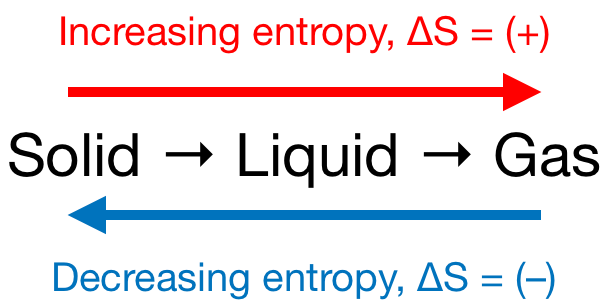# Problem: Which of the following processes would you expect to have a negative value for entropy? NaCl(s) → Na+(aq) + Cl- (aq) H2O(s) →H2O(l) NaCl(s) →NaCl(l) 2 Al(s) + 3Br2(l) →2AlBr3(s) C2H5OH(l) →  C2H4(g) + H2O(g)

###### FREE Expert Solution

We’re being asked to predict the sign of the entropy change (ΔS) for the given reactions. Recall that if:

• ΔS > 0 or ΔS = (+); entropy is increased

• ΔS < 0 or ΔS = (–); entropy is decreased

Remember that phase changes lead to a change in entropy:Also, recall that an increase in the number of gas molecules in the reaction also leads to an increase in entropy.

90% (366 ratings)###### Problem Details

Which of the following processes would you expect to have a negative value for entropy?

NaCl(s) → Na+(aq) + Cl- (aq)

H2O(s) →H2O(l)

NaCl(s) →NaCl(l)

2 Al(s) + 3Br2(l) →2AlBr3(s)

C2H5OH(l) →  C2H4(g) + H2O(g)

Frequently Asked Questions

What scientific concept do you need to know in order to solve this problem?

Our tutors have indicated that to solve this problem you will need to apply the Entropy concept. You can view video lessons to learn Entropy. Or if you need more Entropy practice, you can also practice Entropy practice problems.